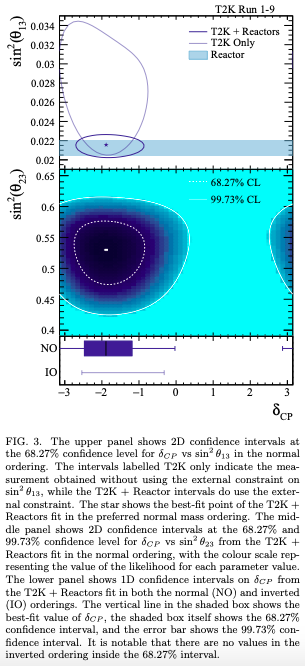## Wednesday, April 15, 2020

### Neutrino Oscillation CP Violation Parameter Measured

Ignore the discussion of the matter-antimatter asymmetry of the universe that is thrown in as a journalistic convention anytime that someone tries to explain CP violation observations in news reporting and in the paper itself as a contextualizing preface. Simply put, even with this observation of near maximal CP violation (with immense uncertainty) the magnitude of the CP violating parameters that are observed in the Standard Model just aren't big enough to produce the existing magnitude of matter and antimatter imbalance from a universe that began in matter-antimatter balance. The paper doesn't claim otherwise, it is simply reporting a measurement of an experimental measurement of a Standard Model fundamental constant.

But, a best ever measurement of one of the least precisely known fundamental constants in the Standard Model is still a big deal, and our understanding of neutrinos remains nebulous in a field where so much is definitively established. The pertinent part of the abstract is as follows:

Until now, the value of δCP has not been substantially constrained by neutrino oscillation experiments. Here we report a measurement using long-baseline neutrino and antineutrino oscillations observed by the T2K experiment that shows a large increase in the neutrino oscillation probability, excluding values of δCP that result in a large increase in the observed antineutrino oscillation probability at three standard deviations (3σ). The 3σ confidence interval for δCP, which is cyclic and repeats every 2π, is [−3.41, −0.03] for the so-called normal mass ordering and [−2.54, −0.32] for the inverted mass ordering.
From here via Science Daily.

The key result from the body text of the paper (in radians) is as follows:
We find the data shows a preference for the normal mass ordering with a posterior probability of 89%, giving a Bayes factor of 8. We find sin2 (θ23) = 0.53+0.03 −0.04 for both mass orderings. Assuming the normal (inverted) mass ordering we find ∆m2 32 = (2.45 ± 0.07) × 10^−3 (∆m2 13 = (2.43±0.07)×10^−3 ) eV^2 /c^4. For δCP our best fit value and 68% (1σ) uncertainties assuming the normal (inverted) mass ordering are −1.89 +0.70 −0.58 (−1.38 +0.48 −0.54), with statistical uncertainty dominating. Our data show a preference for values of δCP which are near maximal CP violation (see Figure 3), while both CP conserving points, δCP = 0 and δCP = π, are ruled out at T2K measurement . Here, we also produce 99.73% (3σ) confidence and credible intervals on δCP . In the normal ordering the interval contains [−3.41,−0.03] (excluding 46% of the range of parameter space), while in the inverted ordering the interval contains [-2.54,-0.32] (excluding 65% of the parameter space). The 99.73% credible interval marginalized across both mass orderings contains [−3.48,0.13] (excluding 42% of the parameter space). The CP-conserving points are not both excluded at the 99.73% level. However, this is the first time closed 99.73% (3σ) intervals on the CP-violating phase δCP have been reported (taking into account both mass orderings) and a large range of values around +π/2 are excluded.
Figure 3 referenced above is this one:Expressed differently, the best fit value is -0.60π + 0.22π -0.18π. This is consistent at a one standard deviation level with maximal CP violation which is -0.50π.

This result is in line with previous results from the T2K experiment. In July of 2018 it found that: "The best fit value for a CP violating phase based upon the latest T2K measurements is -0.6π. Maximal CP violation is -0.5π. Zero CP violation (zero or pi) is excluded at more than two sigma significance."

The press release in Science Daily explains that:

To get the new result, the team fired beams of muon neutrinos and antineutrinos from the J-PARC facility at Tokai, Japan, and detected how many electron neutrinos and antineutrinos arrived at the Super-Kamiokande detector 295km away.
They looked for differences in how the neutrinos or antineutrinos changed flavour, finding neutrinos appear to be much more likely to change than antineutrinos.
The available data also strongly discount the possibility that neutrinos and antineutrinos are as just likely as each other to change flavour. Dr Dunne said: "What our result shows is that we're more than 95 per cent sure that matter neutrinos and antineutrinos behave differently. This is big news in itself; however we do already know of other particles that have matter-antimatter differences that are too small to explain our matter-dominated universe.
Astronomy observations favor a "normal ordering" for the neutrino masses even more strongly than the 89% preference for this derived from oscillation data alone by the T2K experiment. See, e.g., this March 18, 2020 review paper summarizing constraints on neutrino properties from multiple sources rather than a single experiment which shows a 3.5 standard deviation preference for "normal ordering" over an "inverted ordering" of the neutrino masses.

The newly published paper is: Abe, K., Akutsu, R., Ali, A. et al., "Constraint on the matter–antimatter symmetry-violating phase in neutrino oscillations." Nature (April 15, 2020). DOI: 10.1038/s41586-020-2177-0. The open access full text pre-print of this paper was released in October of 2019.

N.B. It is worth noting that the particular numerical values reported above are convention dependent, which means that they depend upon who you define the parameters of the PMNS matrix of which this phase is one of four, although the physical consequences are the same without regard to which parameterization convention is used.